Download Presentation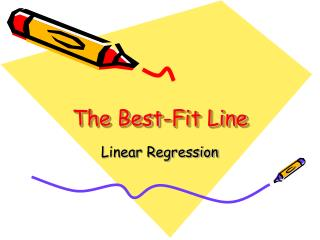The Best-Fit Line

# The Best-Fit Line - PowerPoint PPT Presentation

The Best-Fit Line. Linear Regression. How do you determine the best-fit line through data points?. Fortunately technology, such as the graphing calculator and Excel, can do a better job than your eye and a ruler!. y-variable. x-variable. The Equation of a Straight Line. y = mx + bI am the owner, or an agent authorized to act on behalf of the owner, of the copyrighted work described.
Download Presentation## The Best-Fit Line

An Image/Link below is provided (as is) to download presentation

Download Policy: Content on the Website is provided to you AS IS for your information and personal use and may not be sold / licensed / shared on other websites without getting consent from its author.While downloading, if for some reason you are not able to download a presentation, the publisher may have deleted the file from their server.

- - - - - - - - - - - - - - - - - - - - - - - - - - E N D - - - - - - - - - - - - - - - - - - - - - - - - - -
Presentation Transcript### The Best-Fit Line

Linear RegressionHow do you determine the best-fit line through data points?

Fortunately technology,

such as the graphing calculator

and Excel, can do a better

job than your eye and a ruler!

y-variable

x-variable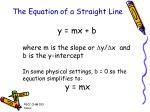The Equation of a Straight Line

y = mx + b

where m is the slope or Dy/Dx and b is the y-intercept

In some physical settings, b = 0 so the equation simplifies to:

y = mx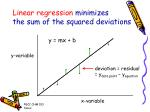Linear regression minimizes

the sum of the squared deviations

y = mx + b

y-variable

deviation = residual

= ydata point – yequation

x-variable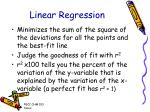Linear Regression
• Minimizes the sum of the square of the deviations for all the points and the best-fit line
• Judge the goodness of fit with r2
• r2 x100 tells you the percent of the variation of the y-variable that is explained by the variation of the x-variable (a perfect fit has r2 = 1)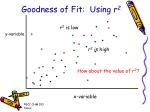r2 is low

r2 is high

Goodness of Fit: Using r2

y-variable

How about the value of r2?

x-variable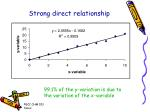Strong direct relationship

99.1% of the y-variation is due to

the variation of the x-variable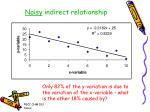Noisy indirect relationship

Only 82% of the y-variation is due to

the variation of the x-variable - what

is the other 18% caused by?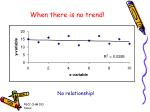When there is no trend!

No relationship!In Excel
• When the chart is active, go to chart, and select Add Trendline, choose the type and on option select display equation and display r2
• For calibration curves, select the set intercept = 0 option Does this make physical sense?Does the set intercept = 0 option

make a difference?

Using the set intercept = 0 option

lowers the r2 value by a small amount

and changes the slope slightlyThe equation becomes

A = mc

or

A = 0.89c

99.1% of the variation of the absorbance is due to the variation of the concentration.